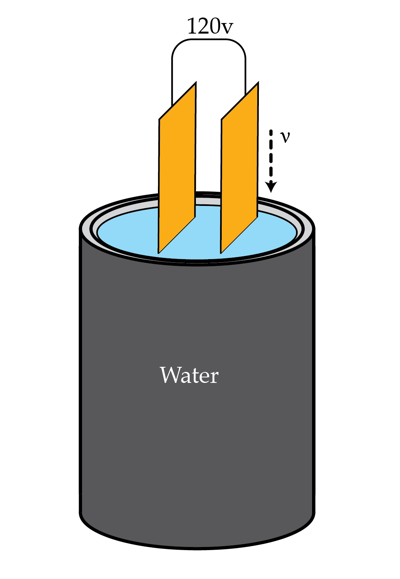# Water and Electricity

A 120V source is connected across the plates of a parallel-plate capacitor. The capacitor is being submerged vertically at constant speed into a container filled with water. What current in Amps flows through the voltage source during this process if it takes $\tau=20 s$ to totally submerge the capacitor? The capacitance (in air) of the capacitor is $C_{0}=12\mu F$ and water's relative permittivity is $\epsilon=81.$Hint: If the capacitor is partially submerged at an instant of time try figuring out how to treat it as two separate capacitors at that instant.

×

Problem Loading...

Note Loading...

Set Loading...